Technical Test SSC JE: Electrical Engineering (EE)- 2

# Technical Test SSC JE: Electrical Engineering (EE)- 2

Test Description

## 100 Questions MCQ Test Mock Test Series for SSC JE Electrical Engineering | Technical Test SSC JE: Electrical Engineering (EE)- 2

Technical Test SSC JE: Electrical Engineering (EE)- 2 for Electrical Engineering (EE) 2022 is part of Mock Test Series for SSC JE Electrical Engineering preparation. The Technical Test SSC JE: Electrical Engineering (EE)- 2 questions and answers have been prepared according to the Electrical Engineering (EE) exam syllabus.The Technical Test SSC JE: Electrical Engineering (EE)- 2 MCQs are made for Electrical Engineering (EE) 2022 Exam. Find important definitions, questions, notes, meanings, examples, exercises, MCQs and online tests for Technical Test SSC JE: Electrical Engineering (EE)- 2 below.
Solutions of Technical Test SSC JE: Electrical Engineering (EE)- 2 questions in English are available as part of our Mock Test Series for SSC JE Electrical Engineering for Electrical Engineering (EE) & Technical Test SSC JE: Electrical Engineering (EE)- 2 solutions in Hindi for Mock Test Series for SSC JE Electrical Engineering course. Download more important topics, notes, lectures and mock test series for Electrical Engineering (EE) Exam by signing up for free. Attempt Technical Test SSC JE: Electrical Engineering (EE)- 2 | 100 questions in 100 minutes | Mock test for Electrical Engineering (EE) preparation | Free important questions MCQ to study Mock Test Series for SSC JE Electrical Engineering for Electrical Engineering (EE) Exam | Download free PDF with solutions
 1 Crore+ students have signed up on EduRev. Have you?
Technical Test SSC JE: Electrical Engineering (EE)- 2 - Question 1

### If induction motor is direct switched-on then it will develop

Technical Test SSC JE: Electrical Engineering (EE)- 2 - Question 2

### Two bulbs 100W, 250 V and 200 W, 250 V are connected in series across a 500V line then-

Technical Test SSC JE: Electrical Engineering (EE)- 2 - Question 3

### Which of the following characteristics of electrons determines the current in a conductor?

Technical Test SSC JE: Electrical Engineering (EE)- 2 - Question 4

The current in the given circuit with a dependent voltage source is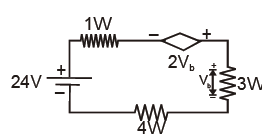Detailed Solution for Technical Test SSC JE: Electrical Engineering (EE)- 2 - Question 4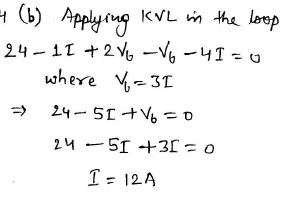Technical Test SSC JE: Electrical Engineering (EE)- 2 - Question 5

Three current i1, i2 and i3 are approaching a mode. If i1=10 sin (400 t + 60º) A and i2 = 10 sin (400 t +60º) A, then i3 is

Detailed Solution for Technical Test SSC JE: Electrical Engineering (EE)- 2 - Question 5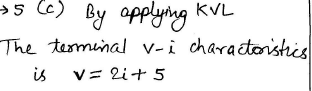Technical Test SSC JE: Electrical Engineering (EE)- 2 - Question 6

The figure shows a network in which the diode is an ideal one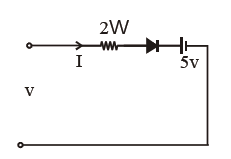The terminal V-I characteristics of the network is given by

Detailed Solution for Technical Test SSC JE: Electrical Engineering (EE)- 2 - Question 6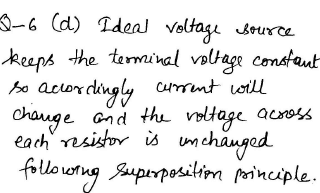Technical Test SSC JE: Electrical Engineering (EE)- 2 - Question 7

In a network made up of linear resistors and ideal voltage sources, values of all resistors are doubled. Then the voltage across each resistor is

Detailed Solution for Technical Test SSC JE: Electrical Engineering (EE)- 2 - Question 7

Even on changing the values of linear resistors, the voltage remains constant in case of ideal voltage source.

Technical Test SSC JE: Electrical Engineering (EE)- 2 - Question 8

Consitor the following circuit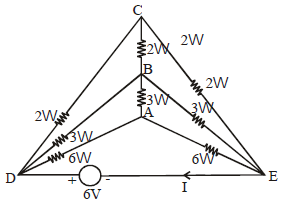What is the value of the current I in the above circuit?

Detailed Solution for Technical Test SSC JE: Electrical Engineering (EE)- 2 - Question 8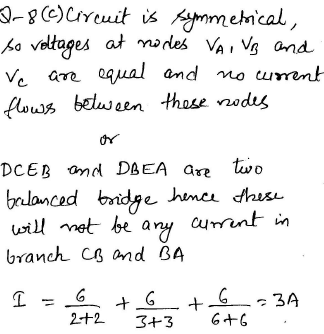Technical Test SSC JE: Electrical Engineering (EE)- 2 - Question 9

For the circuit shown in the given figure the current through L and the voltage across C2 are respectively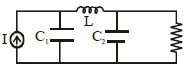Detailed Solution for Technical Test SSC JE: Electrical Engineering (EE)- 2 - Question 9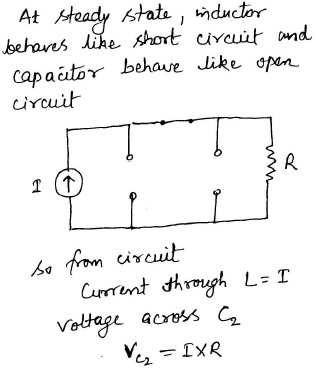Technical Test SSC JE: Electrical Engineering (EE)- 2 - Question 10

A unit impulse voltage is applied at t=0 to the RL circuit shown below while i (0-)= 1A. What isthe expression for i (t)?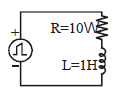Detailed Solution for Technical Test SSC JE: Electrical Engineering (EE)- 2 - Question 10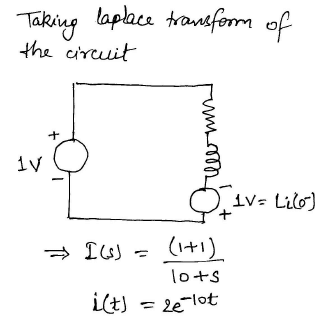Technical Test SSC JE: Electrical Engineering (EE)- 2 - Question 11

It the circuit shown in the figure given below, the switch is opened at t = 0 after having been closed for a long time. What is the current through 50W resistor?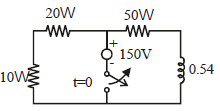Detailed Solution for Technical Test SSC JE: Electrical Engineering (EE)- 2 - Question 11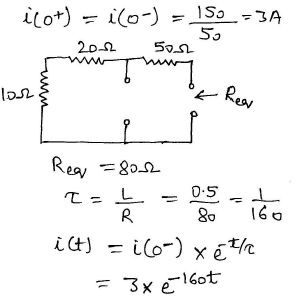Technical Test SSC JE: Electrical Engineering (EE)- 2 - Question 12

For the circuit shown in the given figure the current I is given by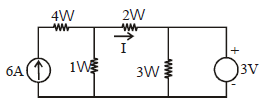Detailed Solution for Technical Test SSC JE: Electrical Engineering (EE)- 2 - Question 12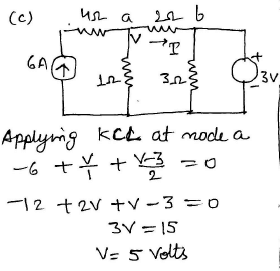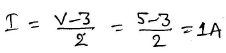Technical Test SSC JE: Electrical Engineering (EE)- 2 - Question 13

The value of V in the circuit shown in the given figure is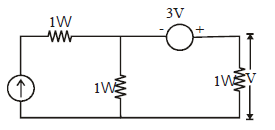where I = 3A

Detailed Solution for Technical Test SSC JE: Electrical Engineering (EE)- 2 - Question 13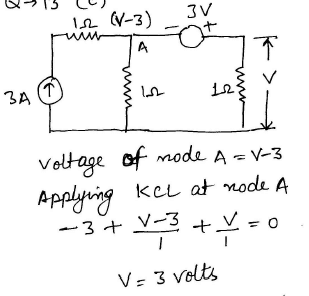Technical Test SSC JE: Electrical Engineering (EE)- 2 - Question 14

Capacitors charge and discharge in __________ manner.

Technical Test SSC JE: Electrical Engineering (EE)- 2 - Question 15

Consider the circuit given below, what is the power delivered by the 24 V source?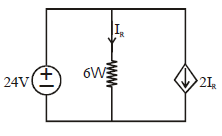Detailed Solution for Technical Test SSC JE: Electrical Engineering (EE)- 2 - Question 15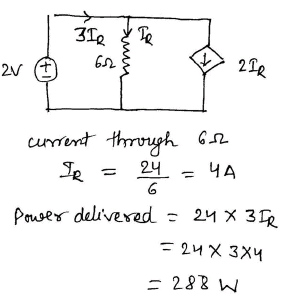Technical Test SSC JE: Electrical Engineering (EE)- 2 - Question 16

A straight conductor of circular x-section carries a current.which one of the following statement is true in this regard?

Technical Test SSC JE: Electrical Engineering (EE)- 2 - Question 17

A cast steel electromagnet has an air gap length of 0.3 cm. Find the ampere turns for the air gap to produce a flux density of 0.7 wb/m2 in the air gap

Detailed Solution for Technical Test SSC JE: Electrical Engineering (EE)- 2 - Question 17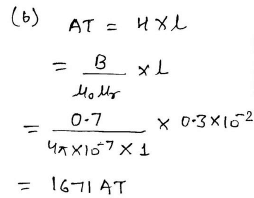Technical Test SSC JE: Electrical Engineering (EE)- 2 - Question 18

A magnetic circuit requires 800 AT to produce a certain quantity of flux in the magnetic circuit. If the excitation coil has 100 turns and 5W resistance, the voltage to be applied in the excitingcoil is

Detailed Solution for Technical Test SSC JE: Electrical Engineering (EE)- 2 - Question 18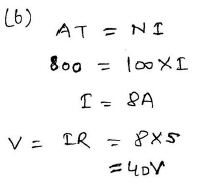Technical Test SSC JE: Electrical Engineering (EE)- 2 - Question 19

The principle of dynamically induced emf is utilised in a

Technical Test SSC JE: Electrical Engineering (EE)- 2 - Question 20

Lower the self inductance of the coil

Technical Test SSC JE: Electrical Engineering (EE)- 2 - Question 21

Two coupled coils connected in series have an equivalent inductance of 16 mH or 8 mH depending on its inter connection. Then the mutual inductance M between the coil is

Detailed Solution for Technical Test SSC JE: Electrical Engineering (EE)- 2 - Question 21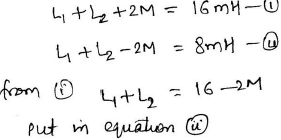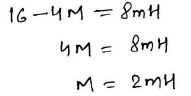Technical Test SSC JE: Electrical Engineering (EE)- 2 - Question 22

The overall inductance of two coils connected in series, with mutual inductance aiding self inductance is L1, with mutual inductance opposing self inductance the overall inductance is L2 . The mutual inductance M is given by

Detailed Solution for Technical Test SSC JE: Electrical Engineering (EE)- 2 - Question 22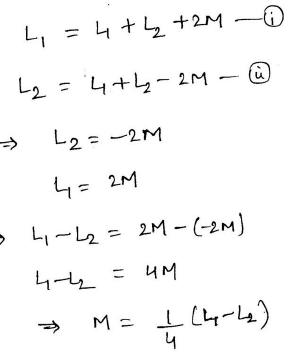Technical Test SSC JE: Electrical Engineering (EE)- 2 - Question 23

Power factor is highest in case of

Technical Test SSC JE: Electrical Engineering (EE)- 2 - Question 24

The purpose of providing choke in a tube light is

Technical Test SSC JE: Electrical Engineering (EE)- 2 - Question 25

Blinking of Fluorescent tube may be on account of

Technical Test SSC JE: Electrical Engineering (EE)- 2 - Question 26

The current drawn by a 220 V dc motor of armature resistance 0.5W and back emf 200V is

Detailed Solution for Technical Test SSC JE: Electrical Engineering (EE)- 2 - Question 26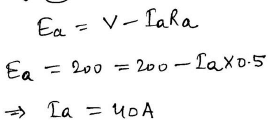Technical Test SSC JE: Electrical Engineering (EE)- 2 - Question 27

A dc shunt generator when driven without connecting field winding shows an open circuit terminal voltage of 12V. When field winding is connected and excited the terminal voltage drop to zero because

Detailed Solution for Technical Test SSC JE: Electrical Engineering (EE)- 2 - Question 27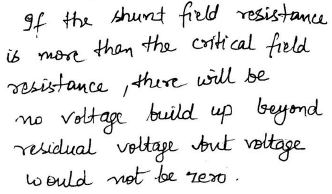Technical Test SSC JE: Electrical Engineering (EE)- 2 - Question 28

A dc series motor is accidently connected to single phase a.c. suply. The torque produced will be

Detailed Solution for Technical Test SSC JE: Electrical Engineering (EE)- 2 - Question 28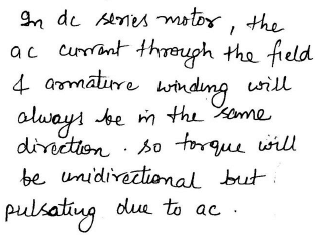Technical Test SSC JE: Electrical Engineering (EE)- 2 - Question 29

At 1200 rpm, the induced emf of a dc machine is 200 V. For an armature current of 15 A the electromagnetic torque produced would be

Detailed Solution for Technical Test SSC JE: Electrical Engineering (EE)- 2 - Question 29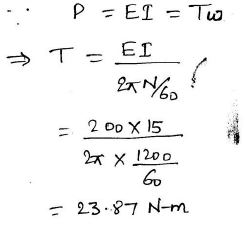Technical Test SSC JE: Electrical Engineering (EE)- 2 - Question 30

Determine the flux per pole for 6 pole dc machine having 240 wave connected conductors, which generates an open circuit voltage of 500 volt.Which is running at 1000 rpm

Detailed Solution for Technical Test SSC JE: Electrical Engineering (EE)- 2 - Question 30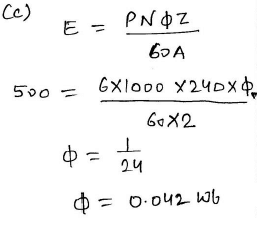Technical Test SSC JE: Electrical Engineering (EE)- 2 - Question 31

The armature resistance of a 6 pole lap wound d.c. machine is 0.05W. If the armature is rewound as a wave winding. What is the armature resistance.

Detailed Solution for Technical Test SSC JE: Electrical Engineering (EE)- 2 - Question 31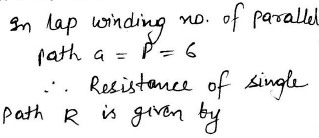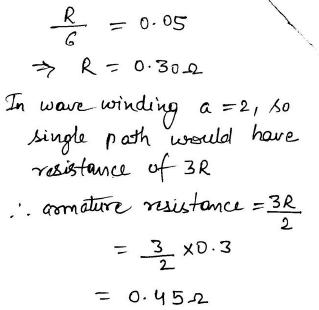Technical Test SSC JE: Electrical Engineering (EE)- 2 - Question 32

A 200/100V, 50Hz transformer is to be excited at 40 Hz. from the 100 V side for the exciting current to remain same the applied voltage should be

Detailed Solution for Technical Test SSC JE: Electrical Engineering (EE)- 2 - Question 32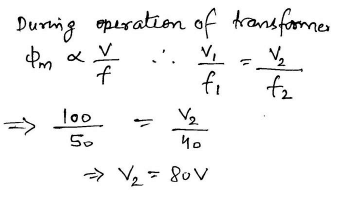Technical Test SSC JE: Electrical Engineering (EE)- 2 - Question 33

Iron losses in a D.C machines are independent of variation in

Technical Test SSC JE: Electrical Engineering (EE)- 2 - Question 34

As a result of armature reaction, the reduction in the total mutual air gap flux in a DC generator is approximately

Technical Test SSC JE: Electrical Engineering (EE)- 2 - Question 35

Which motor has the poorest speed control?

Technical Test SSC JE: Electrical Engineering (EE)- 2 - Question 36

Which of the following application requires high starting torque?

Technical Test SSC JE: Electrical Engineering (EE)- 2 - Question 37

For constant torque drive which speed control method is preferred?

Technical Test SSC JE: Electrical Engineering (EE)- 2 - Question 38

A 100 VA, 120/12V transformer is to be connected so as to form a step-up transformer. A primary voltage of 120V is applied to the transformer. What is the secondary voltage of the transformer?

Detailed Solution for Technical Test SSC JE: Electrical Engineering (EE)- 2 - Question 38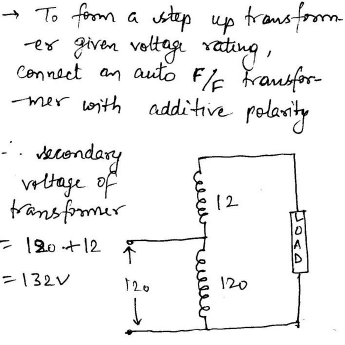Technical Test SSC JE: Electrical Engineering (EE)- 2 - Question 39

The synchronizing reactance of a 500V 50 KVA alternator having an effective resistance of 0.2W, if an excitation current of 10 A produces 200A armature current on short circuit and an emf of 450 volts on open circuit is

Detailed Solution for Technical Test SSC JE: Electrical Engineering (EE)- 2 - Question 39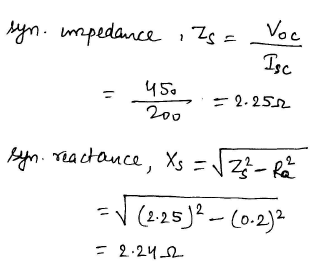Technical Test SSC JE: Electrical Engineering (EE)- 2 - Question 40

When the rotor speed, in a synchronous machine becomes more than the synchronous speed during hunting, the damper boars develop

Technical Test SSC JE: Electrical Engineering (EE)- 2 - Question 41

The starting current of a 3–f induction motor is 5 times the rated current, while the rated slip is 4%. The ratio of starting torque to full load torque is

Detailed Solution for Technical Test SSC JE: Electrical Engineering (EE)- 2 - Question 41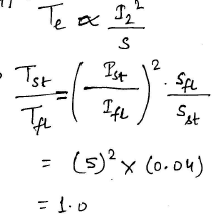Technical Test SSC JE: Electrical Engineering (EE)- 2 - Question 42

Squired cage bars place in the rotor pole faces of an alternator help reducing hunting

Technical Test SSC JE: Electrical Engineering (EE)- 2 - Question 43

A 3-f synchronous motor has

Technical Test SSC JE: Electrical Engineering (EE)- 2 - Question 44

Which of the following device can be used as a phase advancer?

Technical Test SSC JE: Electrical Engineering (EE)- 2 - Question 45

Capacitor in a single phase induction motor is used for

Technical Test SSC JE: Electrical Engineering (EE)- 2 - Question 46

The repulsion start induction motor has the advantage of

Technical Test SSC JE: Electrical Engineering (EE)- 2 - Question 47

Single phase induction motor built with a variable air gap and without dc excitation is called____ motor

Technical Test SSC JE: Electrical Engineering (EE)- 2 - Question 48

A hysteresis motor

Technical Test SSC JE: Electrical Engineering (EE)- 2 - Question 49

Which of the following motors are used in largest number?

Technical Test SSC JE: Electrical Engineering (EE)- 2 - Question 50

A Buchholz relay is used for

Technical Test SSC JE: Electrical Engineering (EE)- 2 - Question 51

Which type of connection is employed a for current transformers for the protection of stardelta connected 3–f transformer?

Detailed Solution for Technical Test SSC JE: Electrical Engineering (EE)- 2 - Question 51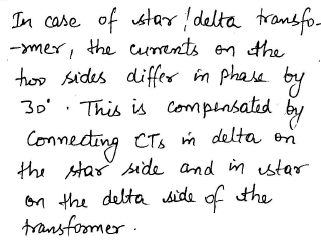Technical Test SSC JE: Electrical Engineering (EE)- 2 - Question 52

Which circuit breaker is preferred to be installed in extra high voltage AC system?

Technical Test SSC JE: Electrical Engineering (EE)- 2 - Question 53

A generating station has a maximum demand of 30 MW, a load factor of 60% and a plant capacity factor of 50%. The reserve capacity of the plant is

Detailed Solution for Technical Test SSC JE: Electrical Engineering (EE)- 2 - Question 53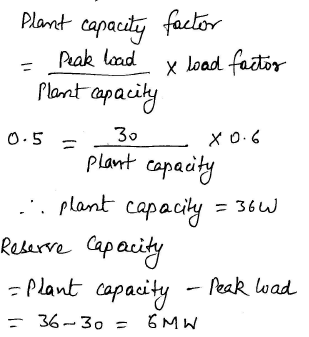Technical Test SSC JE: Electrical Engineering (EE)- 2 - Question 54

The rating of fuse wire is always expressed in

Technical Test SSC JE: Electrical Engineering (EE)- 2 - Question 55

Transmission of power by ac cables is impossible beyond.

Technical Test SSC JE: Electrical Engineering (EE)- 2 - Question 56

Earth wire should be

Technical Test SSC JE: Electrical Engineering (EE)- 2 - Question 57

Low power factor is usually not due to

Technical Test SSC JE: Electrical Engineering (EE)- 2 - Question 58

A power station has a maximum demand of 15000 KW. The annual load factor is 50% and plant capacity factor is 40%. What is the reserve capacity of the plant?

Detailed Solution for Technical Test SSC JE: Electrical Engineering (EE)- 2 - Question 58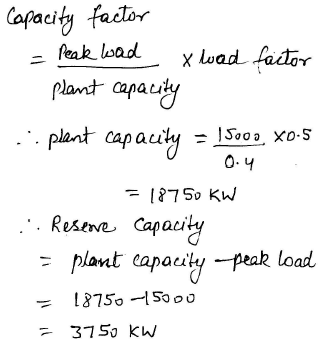Technical Test SSC JE: Electrical Engineering (EE)- 2 - Question 59

If all the sequence voltages at the fault point in a power system are equal, then the fault is a

Detailed Solution for Technical Test SSC JE: Electrical Engineering (EE)- 2 - Question 59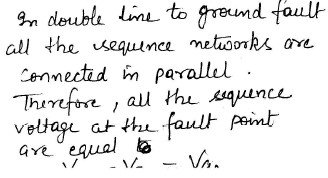Technical Test SSC JE: Electrical Engineering (EE)- 2 - Question 60

A 10 kVA, 400 V/200V single phase transformer with 10% impedance, draws a steady shor tcircuit current of

Detailed Solution for Technical Test SSC JE: Electrical Engineering (EE)- 2 - Question 60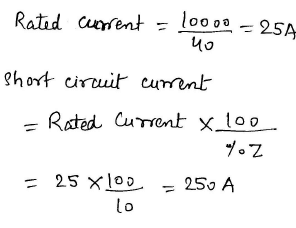Technical Test SSC JE: Electrical Engineering (EE)- 2 - Question 61

The breakdown voltage of a cable depends upon

Technical Test SSC JE: Electrical Engineering (EE)- 2 - Question 62

As per electricity regulations in India the maximum permissible voltage change at consumer premises should be

Technical Test SSC JE: Electrical Engineering (EE)- 2 - Question 63

On what basis is the insulation level of a 400 KV, EHV overhead transmission line decided?

Technical Test SSC JE: Electrical Engineering (EE)- 2 - Question 64

Series capacitors can be used in distribution lines

Technical Test SSC JE: Electrical Engineering (EE)- 2 - Question 65

Merz-price protection is employed for protection of

Technical Test SSC JE: Electrical Engineering (EE)- 2 - Question 66

Impulse ratios of insulator and lightening arrestor should be

Technical Test SSC JE: Electrical Engineering (EE)- 2 - Question 67

In a thyrite lightning arrester the resistance

Technical Test SSC JE: Electrical Engineering (EE)- 2 - Question 68

Technical Test SSC JE: Electrical Engineering (EE)- 2 - Question 69

For quick speed reversal the motor preferred is

Technical Test SSC JE: Electrical Engineering (EE)- 2 - Question 70

Ward-leonard controlled dc drives are usually used for _____ duty excavators

Technical Test SSC JE: Electrical Engineering (EE)- 2 - Question 71

The underground system cannot be operated above

Technical Test SSC JE: Electrical Engineering (EE)- 2 - Question 72

An instrument which detects electric current is known as

Technical Test SSC JE: Electrical Engineering (EE)- 2 - Question 73

Which of the following transducer is classified as an active transducer?

Technical Test SSC JE: Electrical Engineering (EE)- 2 - Question 74

A first order instrument is characterized by

Technical Test SSC JE: Electrical Engineering (EE)- 2 - Question 75

Which of the following instrument will be used to measure a small current of very high frequency?

Detailed Solution for Technical Test SSC JE: Electrical Engineering (EE)- 2 - Question 75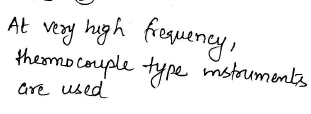Technical Test SSC JE: Electrical Engineering (EE)- 2 - Question 76

Which one of the following passive components has the most precise and accurate standard?

Technical Test SSC JE: Electrical Engineering (EE)- 2 - Question 77

An ac voltmeter using full wave rectification and having sinusoidal input has an ac sensitivity equal to

Detailed Solution for Technical Test SSC JE: Electrical Engineering (EE)- 2 - Question 77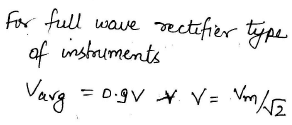Technical Test SSC JE: Electrical Engineering (EE)- 2 - Question 78

A spring controlled moving iron voltmeter draws a current of 1mA for full scale value of 100V. If it draws a current of 0.5 mA, the meter reading is

Detailed Solution for Technical Test SSC JE: Electrical Engineering (EE)- 2 - Question 78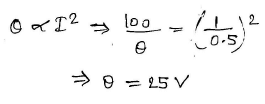Technical Test SSC JE: Electrical Engineering (EE)- 2 - Question 79

By mistake, an ammeter is used as a voltmeter. In all probabilities, it will

Detailed Solution for Technical Test SSC JE: Electrical Engineering (EE)- 2 - Question 79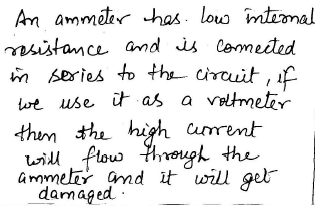Technical Test SSC JE: Electrical Engineering (EE)- 2 - Question 80

Technical Test SSC JE: Electrical Engineering (EE)- 2 - Question 81

A standard resistance is made 'Bifilar' type to eliminate

Technical Test SSC JE: Electrical Engineering (EE)- 2 - Question 82

Maxwell's inductance capacitance bridge is used for measurement of inductance of

Technical Test SSC JE: Electrical Engineering (EE)- 2 - Question 83

Electrostatic type instruments are mainly used for measurement of:

Technical Test SSC JE: Electrical Engineering (EE)- 2 - Question 84

In a two wattmeter method of measuring 3-f power, the wattmeter indicate equal and opposite readings. When load power factor is

Detailed Solution for Technical Test SSC JE: Electrical Engineering (EE)- 2 - Question 84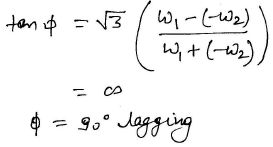Technical Test SSC JE: Electrical Engineering (EE)- 2 - Question 85

In eddy currents damping system, the disc employed should be of

Technical Test SSC JE: Electrical Engineering (EE)- 2 - Question 86

If the instrument has square law response, it can be used for the measurement of

Technical Test SSC JE: Electrical Engineering (EE)- 2 - Question 87

Error due to hysteresis is predominant in

Technical Test SSC JE: Electrical Engineering (EE)- 2 - Question 88

To measure current in the MHz range we use instrument of

Technical Test SSC JE: Electrical Engineering (EE)- 2 - Question 89

The shunt type ohmmeter is not suitable for high resistance measurement because

Technical Test SSC JE: Electrical Engineering (EE)- 2 - Question 90

Ebers - model of a transistor represents two diodes

Technical Test SSC JE: Electrical Engineering (EE)- 2 - Question 91

In an N-P-N transistor, the leakage current is due to

Technical Test SSC JE: Electrical Engineering (EE)- 2 - Question 92

The action of JFET in its equivalent circuit can best be represented as a

Technical Test SSC JE: Electrical Engineering (EE)- 2 - Question 93

In JFET drain current is maximum when VGS is

Technical Test SSC JE: Electrical Engineering (EE)- 2 - Question 94

The diffusion current is proportional to

Technical Test SSC JE: Electrical Engineering (EE)- 2 - Question 95

For highly doped diode

Technical Test SSC JE: Electrical Engineering (EE)- 2 - Question 96

The concentration of minority carriers in an extrinsic semiconductor under equilibrium is

Technical Test SSC JE: Electrical Engineering (EE)- 2 - Question 97

Bridge Rectifiers are preferred because

Detailed Solution for Technical Test SSC JE: Electrical Engineering (EE)- 2 - Question 97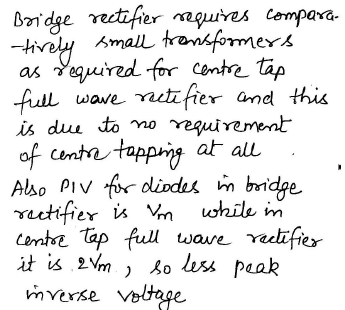Technical Test SSC JE: Electrical Engineering (EE)- 2 - Question 98

A photodiode works on the principle of

Technical Test SSC JE: Electrical Engineering (EE)- 2 - Question 99

In a bridge a.c. to d.c converter using p-n diodes , if the input voltage is Vsinωt what is the peak inverse voltage across any diode?

Detailed Solution for Technical Test SSC JE: Electrical Engineering (EE)- 2 - Question 99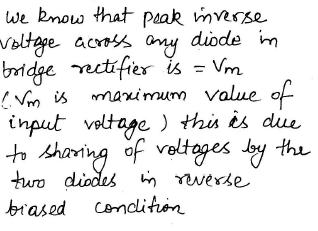Technical Test SSC JE: Electrical Engineering (EE)- 2 - Question 100

​Early effect in BJT refers to

Detailed Solution for Technical Test SSC JE: Electrical Engineering (EE)- 2 - Question 100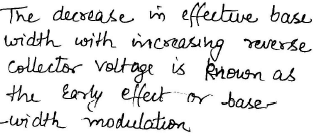## Mock Test Series for SSC JE Electrical Engineering

2 videos|1 docs|55 tests
 Use Code STAYHOME200 and get INR 200 additional OFF Use Coupon Code
Information about Technical Test SSC JE: Electrical Engineering (EE)- 2 Page
In this test you can find the Exam questions for Technical Test SSC JE: Electrical Engineering (EE)- 2 solved & explained in the simplest way possible. Besides giving Questions and answers for Technical Test SSC JE: Electrical Engineering (EE)- 2, EduRev gives you an ample number of Online tests for practice

## Mock Test Series for SSC JE Electrical Engineering

2 videos|1 docs|55 tests